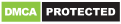# Fractions – calculate and compare

### Additional Information

Genres
Version
-
Developer
-
Requires
-
Updated
-Free offline fraction calculator ✌, supporting also decimals, percentages, parentheses and powers. Shows a detailed solution for all calculations. 😊

At school 🏫 or home 🏠 need to solve simple or complex math fraction problems, convert fractions into decimals, or decimals into fractions? – this fraction calculator will do it ✅ and will show how it was done 😺

Perfect for students, parents, teachers, and woodworkers to solve or verify fraction everyday problems.

Features of this fraction calculator free app ➕ ➖ ❌ ➗

🍉 Supports combining decimal and fractional numbers. Automatically reduces fraction results to its simplest form.

🍇 Converts fractions into decimals and decimals into fractions. Calculated result is displayed using fractions. Equivalent value in decimals is also available.

🍌 Shows a detailed solution, step by step for all fraction problems and calculations.

🍎 A history of previous calculations is available.

🍒 Besides basic math operations such as add, subtract, multiply and divide; fraction calculator allows calculating percentages for fractions.

🍅 Triple keypad available

🍍 Works with big numbers. No limits 😋

🍑 Compare fractions.

We have made this fraction calculator app available in multiple colors 🌈 for you!

© UUCMobile, 2020
Mixed fractions and improper fractions.

Recommended for you

You may also like

Comments